You are currently offline. Some features of the site may not work correctly.

# Covering graph

Known as: Covering
In the mathematical discipline of graph theory, a graph C is a covering graph of another graph G if there is a covering map from the vertex set of C… Expand
Wikipedia

## Papers overview

Semantic Scholar uses AI to extract papers important to this topic.
2018
2018
• Des. Codes Cryptogr.
• 2018
• Corpus ID: 52909182
A covering array $$\text{ CA }(N;t,k,v)$$CA(N;t,k,v) is an $$N\times k$$N×k array such that in every $$N\times t$$N×t subarray… Expand
Is this relevant?
2009
2009
• Eur. J. Comb.
• 2009
• Corpus ID: 30846283.oa
In this paper we study the spectral structure of the discrete Laplacian on an infinite graph. We show that, for a finite graph… Expand
Is this relevant?
2009
2009
• Theor. Comput. Sci.
• 2009
• Corpus ID: 41178120.oa
We consider computational problems on covering graphs with bicliques (complete bipartite subgraphs). Given a graph and an integer… Expand
Is this relevant?
2005
2005
• J. Comb. Theory, Ser. B
• 2005
• Corpus ID: 18410450.oa
We study the problem of covering graphs with trees and a graph of bounded maximum degree. By a classical theorem of Nash-Williams… Expand
Is this relevant?
Highly Cited
2005
Highly Cited
2005
• ArXiv
• 2005
• Corpus ID: 60026
The goal of the present paper is the derivation of a framework for the finite-length analysis of message-passing iterative… Expand
•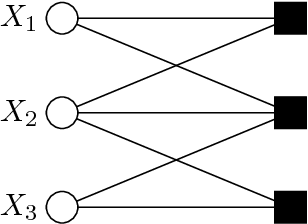•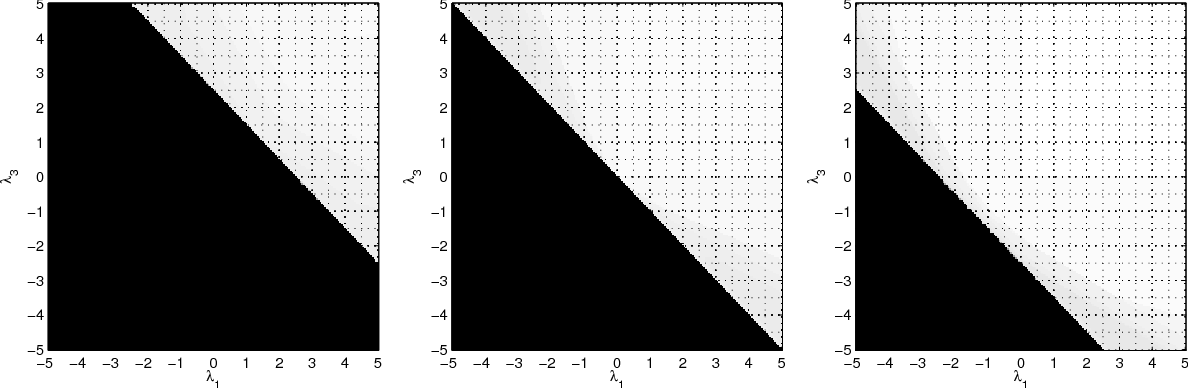•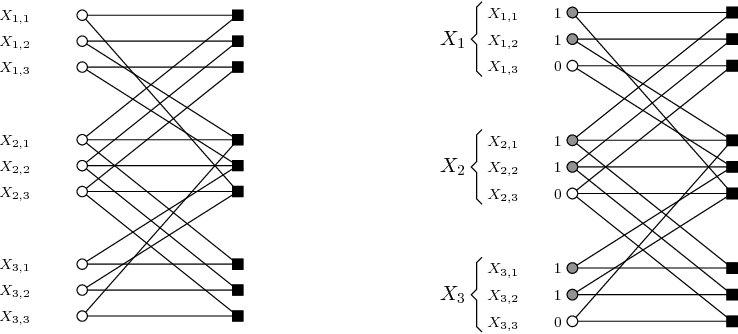•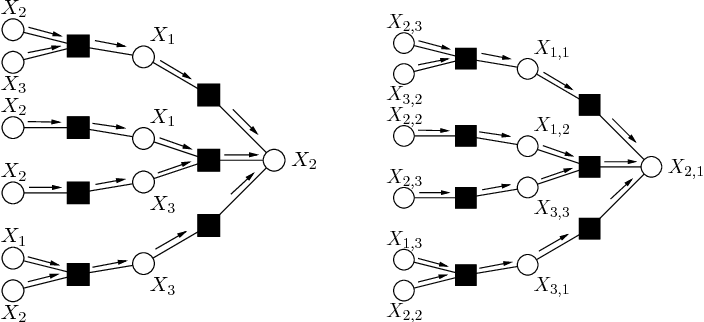•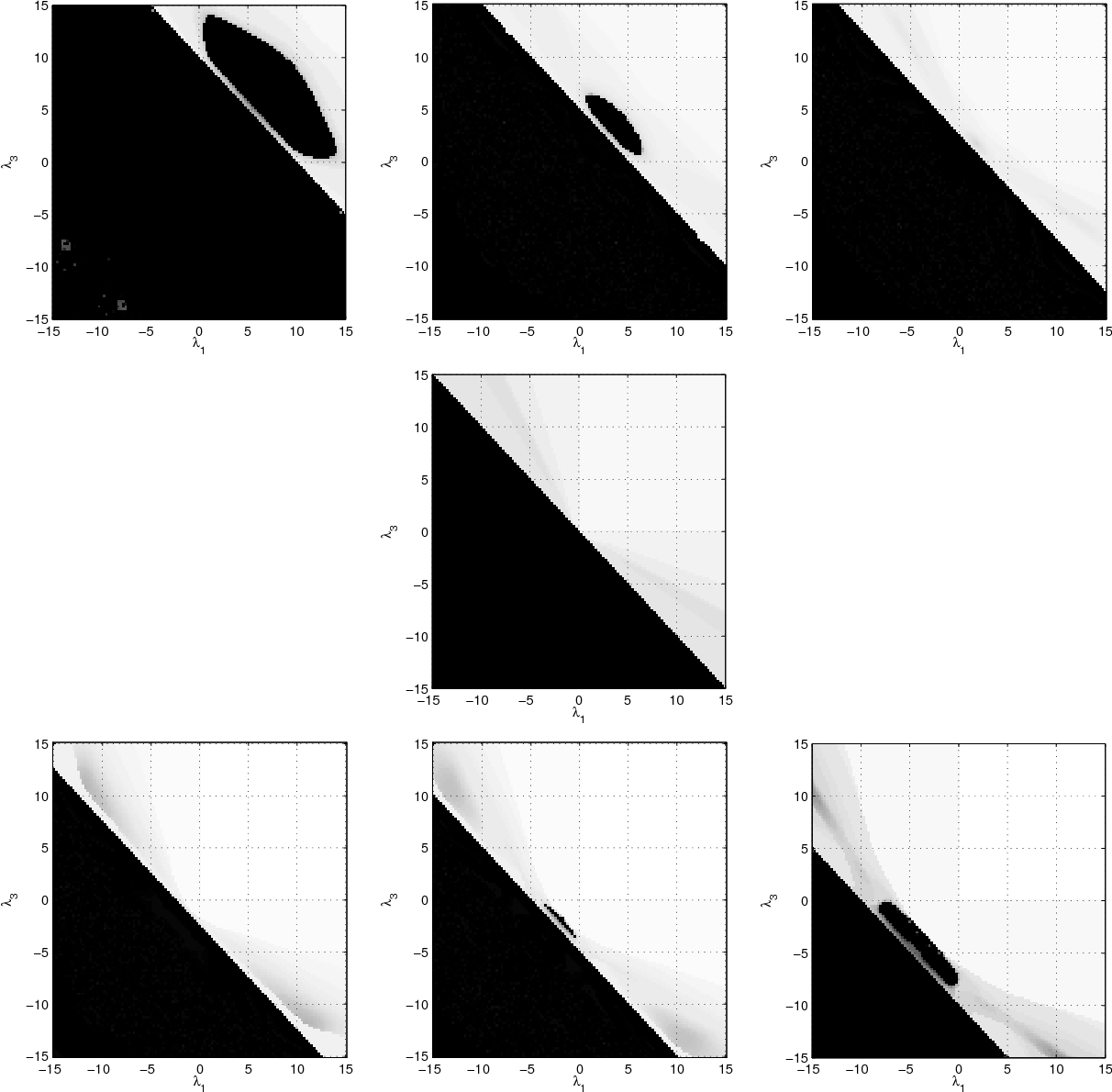Is this relevant?
2005
2005
• Eur. J. Comb.
• 2005
• Corpus ID: 29878870
A graph may be the Kronecker cover in more than one way. In this note we explore this phenomenon and apply it to show that the… Expand
•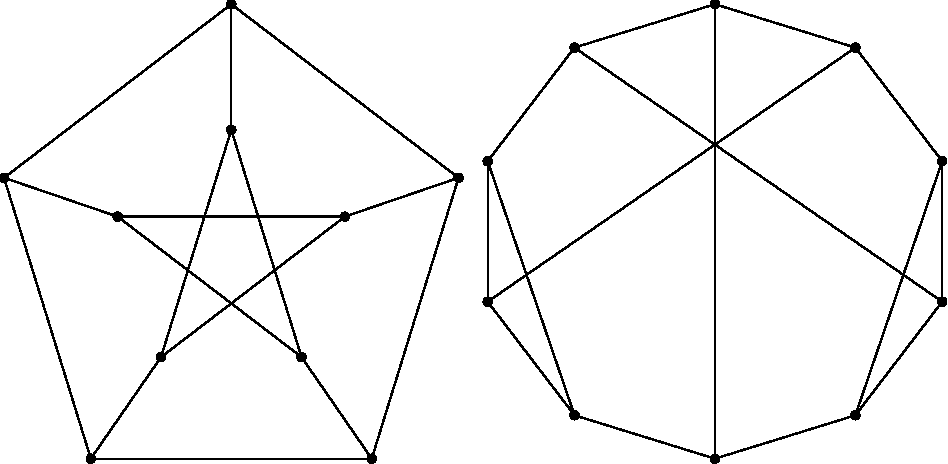•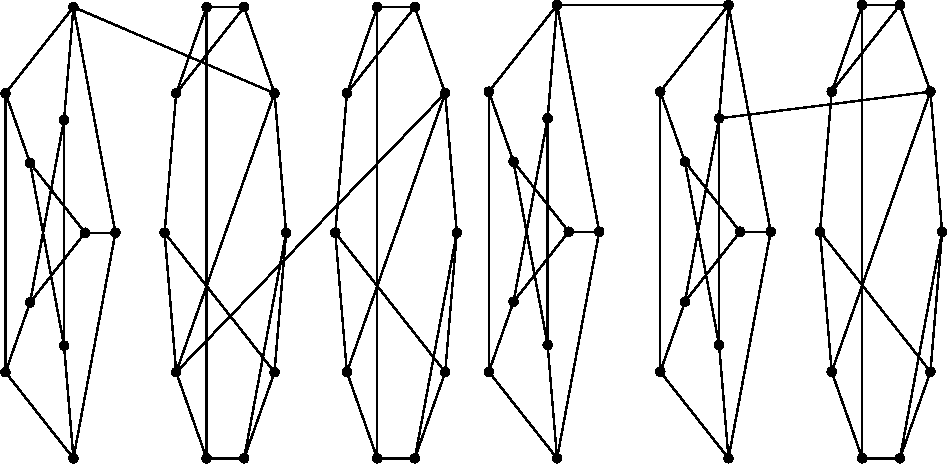Is this relevant?
Highly Cited
2003
Highly Cited
2003
• 2003
• Corpus ID: 16913204
Codewords in finite covers of a Tanner graph G are characterized. Since iterative, locally operating decoding algorithms cannot… Expand
•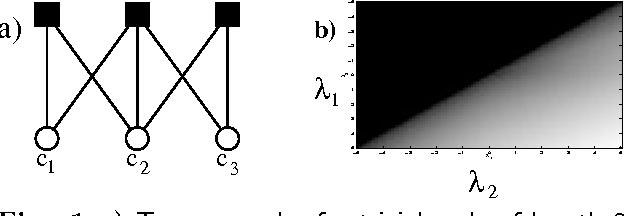•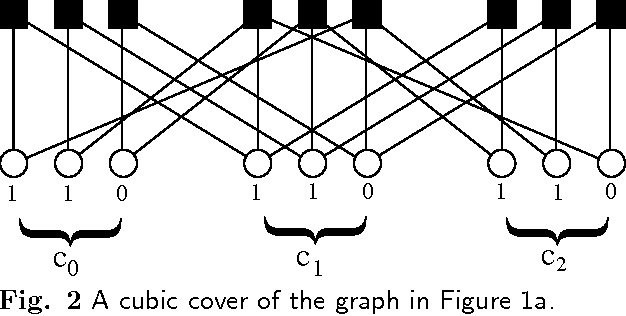•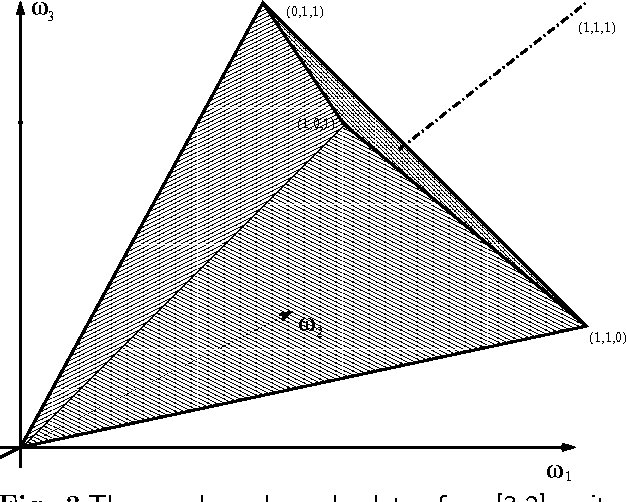•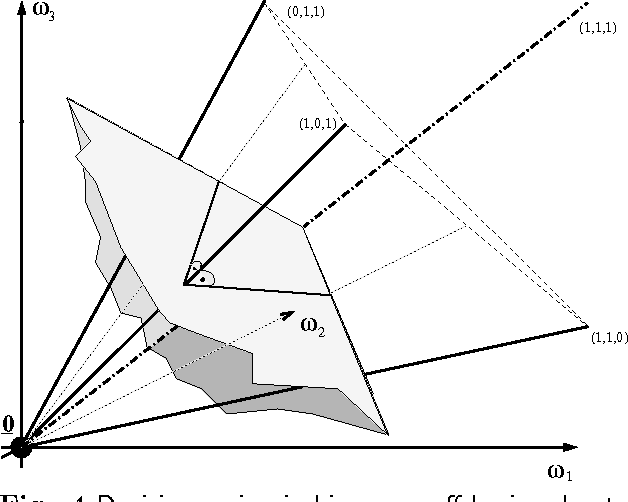•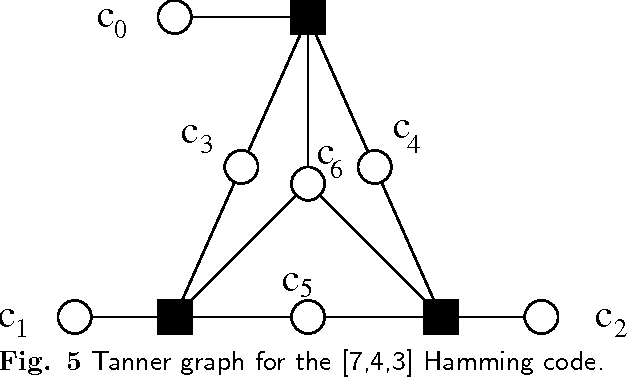Is this relevant?
2000
2000
• Discret. Math.
• 2000
• Corpus ID: 17462310.oa
Abstract In this paper we study when a bipartite graph is a covering of a non-bipartite graph. We give a characterization of all… Expand
Is this relevant?
1994
1994
Abstract We consider the undirected covering graph G of a finite (meet) semilattice X endowed with a lower valuation. More… Expand
Is this relevant?
1984
1984
As is well known the covering graph ( = Hasse diagram) of any median semilattice is a median graph, and every median graph is the… Expand
•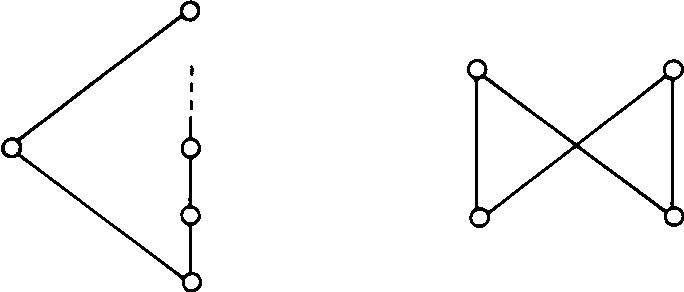•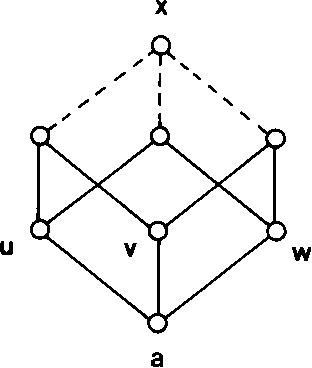Is this relevant?